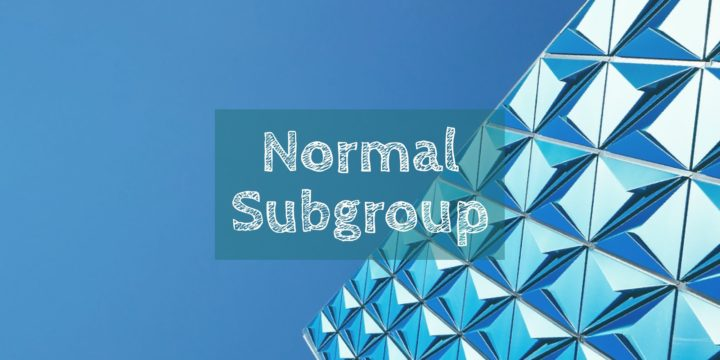# Union of Two Subgroups is Not a Group## Problem 625

Let $G$ be a group and let $H_1, H_2$ be subgroups of $G$ such that $H_1 \not \subset H_2$ and $H_2 \not \subset H_1$.

(a) Prove that the union $H_1 \cup H_2$ is never a subgroup in $G$.

(b) Prove that a group cannot be written as the union of two proper subgroups.Add to solve later

## Proof.

### Prove that the union $H_1 \cup H_2$ is never a subgroup in $G$.

Seeking a contradiction, let us assume that the union $H_1 \cup H_2$ is a subgroup of $G$.
Since $H_1 \not \subset H_2$, there exists an element $a\in H_1$ such that $a\notin H_2$.
Similarly, as $H_2 \not \subset H_1$, there exists an element $b\in H_2$ such that $b\notin H_1$.

As we are assuming $H_1 \cup H_2$ is a group, we have $ab\in H_1 \cup H_2$.
It follows that either $ab \in H_1$ or $ab \in H_2$.

If $ab \in H_1$, then we have
$b=a^{-1}(ab) \in H_1$ as both $a^{-1}$ and $ab$ are elements in the subgroup $H_1$.
This contradicts our choice of the element $b$.

Similarly, if $ab \in H_2$, we have
$a=(ab)b^{-1} \in H_2,$ which contradicts the choice of $a$.

In either case, we reached a contradiction.
Thus, we conclude that the union $H_1 \cup H_2$ is not a subgroup of $G$.

### (b) Prove that a group cannot be written as the union of two proper subgroups.

This is a special case of part (a).

If a group $G$ is a union of two proper subgroup $H_1$ and $H_2$, then we must have $H_1 \not \subset H_2$ and $H_2 \not \subset H_1$, otherwise $G=H_1$ or $G=H_2$ and this is impossible as $H_1, H_2$ are proper subgroups.
Then $G=H_1\cup H_2$ is a subgroup of $G$, which is prohibited by part (a).

Thus, any group cannot be a union of proper subgroups.Add to solve later

### More from my site

#### You may also like...

This site uses Akismet to reduce spam. Learn how your comment data is processed.

###### More in Group Theory##### Normal Subgroup Whose Order is Relatively Prime to Its Index

Let $G$ be a finite group and let $N$ be a normal subgroup of $G$. Suppose that the order $n$...

Close